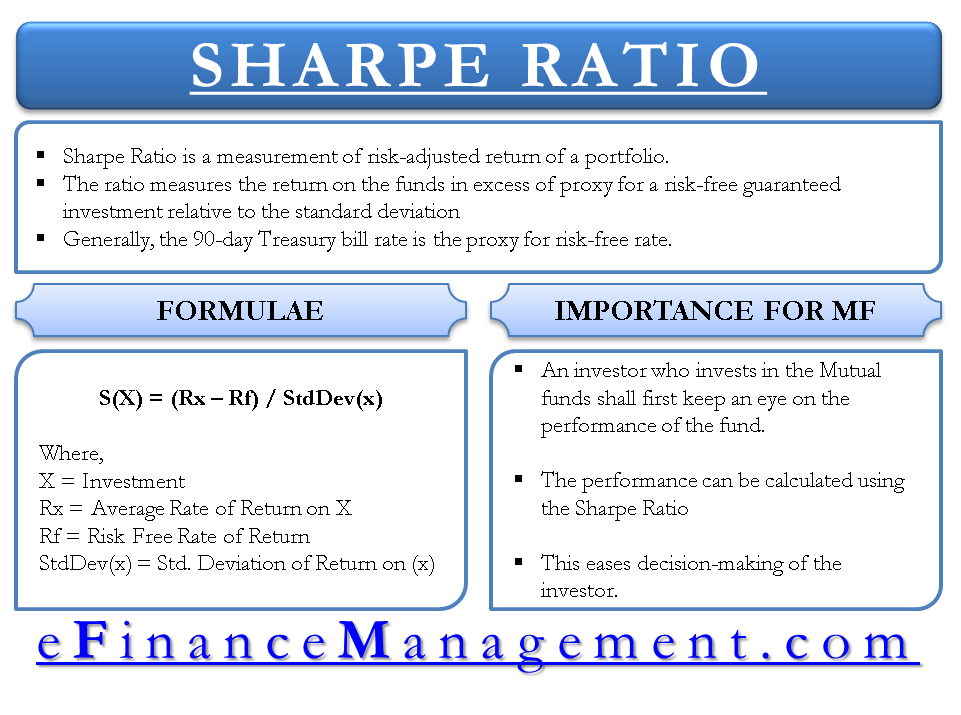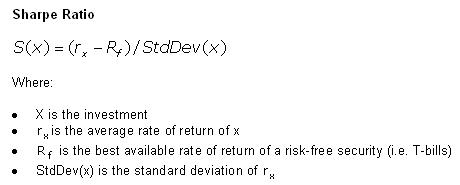# Sharpe Ratio

## What is Sharpe Ratio?

Sharpe Ratio is a measurement of risk-adjusted return of a portfolio. The concept is named after William F. Sharpe of Stanford University. The ratio measures the return on the funds in excess of proxy for a risk-free guaranteed investment relative to the standard deviation. Generally, the 90-day Treasury bill rate is the proxy for risk-free rate.

A portfolio with higher Sharpe ratio is superior to its peers. Among the many formulas that are available, mutual fund managers use this ratio to measure the risk-adjusted returns on their portfolio.

## Explaining Sharpe Ratio in Simple Language

The ratio gives the investor an idea of how much extra returns he is earning in the volatile market for holding a riskier asset. The whole idea behind calculating the Sharpe ratio is to know the compensation an investor is getting for the additional risk he is bearing for not holding a risk free asset.Let us have a look at the formula of Sharpe Ratio.

## Sharpe Ratio Formula

William F. Shape developed the Sharpe ratio formula. The formula of Sharpe ratio is as follows.

Sharpe Ratio = (Mean Portfolio Return − Risk-Free Rate) / Standard Deviation of Portfolio ReturnNote: The formula image is taken from Investopedia.

## Example of Sharpe Ratio

Let us understand the Sharpe ratio formula with the help of an example.

• Suppose the financial asset has an expected rate of return of 9%. The risk-free rate is 3%. Calculate the Sharpe ratio when the standard deviation of the asset’s excess return is 9%.

Sharpe Ratio = (0.09 – 0.03) / 0.09 = 0.67

• In another case, a portfolio has an expected rate of return of 13%.  The risk-free rate is 3% and standard deviation of the asset’s excess return is 9%. Calculate Sharpe ratio.

Sharpe Ratio = (0.13 – 0.03) / 0.09 = 1.11

From the above two examples, we can see that the Sharpe ratio is higher in case of the second example. Higher the Sharpe ratio better is the portfolio. Hence, the second portfolio will give higher returns.

Let us now understand the importance of Share ratio formula for Mutual Funds.

## Importance of Share Ratio Formula for Mutual Funds

An investor who is keen to invest in Mutual Funds shall always look at the performance of the funds first. The performance of the funds is measured using the Sharpe ratio. Sharpe ratio gives the investor the exact information about which Mutual Fund has the best performance among the options available. This eases decision-making of the investor. The comparison between different Mutual Funds can be made by checking the Sharpe ratio of the different funds. The Higher ratio represents higher returns for every unit of risk.

Conclusion

Sharpe ratio is one of the most important tools to measure the performance of any fund or investment. The investors get the real benefit using this ratio. Based on the Sharpe ratio, the investor can determine whether the fund meets his requirements or not. The ratio is used all over the globe and investors should use it for their benefit. Sharpe ratio helps in getting the right analysis of the funds and enhancing the returns on investment.

Also learn about other ratios like P/E Ratio, Cash Ratio and Earnings Per Share by clicking on the link.1–4

1.
Kenton W. Sharpe Ratio. Investopedia. January 2019. [Source]
2.
sharpe ratio. economictimes. January 2019. [Source]
3.
Sharpe Ratio. morningstar. January 2019. [Source]
4.
How to Calculate Sharpe Ratio: Definition, Formula & Examples | Study.com. Study.com. January 2019. [Source]
Last updated on : August 28th, 2019
1.Bonnee Prasad